# Physics - What is Joule's Law

What is Joule's Law

Accorrding to Joule's law, the current passing thrrough a resistor produces heat. The quantity of heat produce 'Q' is equal to the work done by the electric field on the free electrons. If the potential differrence between the ends of resistor is 'V' and current 'i' passes through it for 't' sec. The workdone (w) in time 't' is.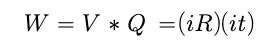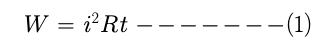This is equal to the heat produced in the resistor due to collision of free electorn with the lattice under the electric field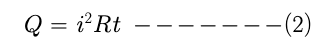1. The heat produced in a given resistor in a given time is propotional to the square of the currrent.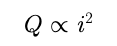2. The heat produced in a given resistor in a given time is propotional to the time for which the currrent exists in it and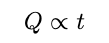3. The heat produced in a given resistor by a given currrent in a given time is proportional to its resistance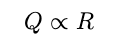Electric heater, electric iron, electric bulb etc are some of the instrument which work on Joule's Law and covert electrical energy to heat energy.

Post By : Rahul Kumar 19 May, 2020 259 views Physics